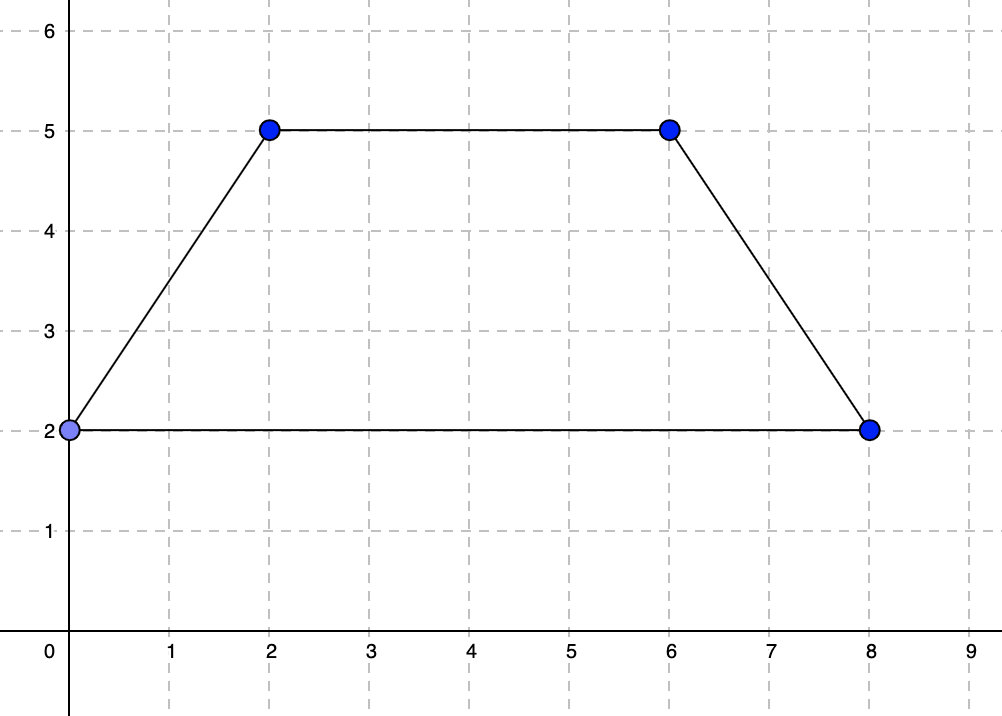시간 제한메모리 제한제출정답맞힌 사람정답 비율
서브태스크 참고 (추가 시간 없음) 1024 MB322100.000%

## 문제

You are given two integers, the number of vertices $N$ and area $A$. You need to construct a simple polygon of $N$ vertices such that the area of the polygon is exactly $\frac{A}{2}$, and all the vertices have non-negative integer coordinates with value up to $10^9$.

A simple polygon is one that:

• Defines a closed area.
• Does not have self-intersections, even at a single point.
• No two consecutive edges form a straight angle.

## 입력

The first line of the input gives the number of test cases, $T$. $T$ lines follow. The first line of each test case contains two integers, $N$ denoting the number of vertices and $A$, denoting double the required area of the polygon.

## 출력

For each test case, output one line containing Case #x: y, where $x$ is the test case number (starting from 1) and $y$ is IMPOSSIBLE if it is not possible to construct a polygon with the given requirements and POSSIBLE otherwise.

If you output POSSIBLE, output $N$ more lines with $2$ integers each. The $i$-th line should contain two integers $X_i$ and $Y_i$ which denote the coordinates of the $i$-th vertex. For each $i$, the coordinates should satisfy the $0 \le X_i,Y_i ≤ 10^9$ constraints. Vertices of the polygon should be listed in consecutive order ($vertex_i$ should be adjacent to $vertex_{i-1}$ and $vertex_{i+1}$ in the polygon).

If there are multiple possible solutions, you can output any of them.

## 제한

• $1 \le T \le 100$.
• $1 \le A \le 10^9$.

## Test Set 1 (15점)

시간 제한: 20 초

• $3 \le N\le 5$.

## Test Set 2 (24점)

시간 제한: 40 초

• $3 \le N \le 1000$.

## 예제 입력 1

2
4 36
5 2


## 예제 출력 1

Case #1: POSSIBLE
2 5
6 5
8 2
0 2
Case #2: IMPOSSIBLEIn Sample Case #1, we can output the above quadrilateral with coordinates $(2,5)$, $(6,5)$, $(0,2)$ and $(8,2)$. The area of this quadrilateral is equal to $18$.

In Sample Case #2, there is no way to construct a polygon with $5$ vertices and area equal to $1$.

## 채점 및 기타 정보

• 예제는 채점하지 않는다.